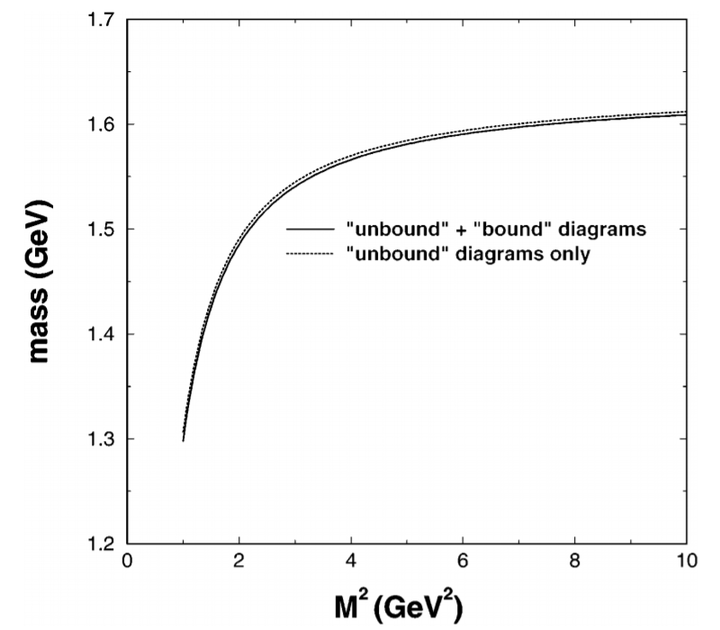# Multiquark picture for Σ (1620)### Abstract

In this work we report on a new QCD sum rule analysis to predict masses of the excited baryon states (e.g. Σ (1620) and Λ (1405)) by using multiquark interpolating fields ((q¯q)(qqq)). For the Σ (1620) we consider the ¯KN, πΣ, and πΛ (I = 1) multiquark interpolating fields. The calculated mass from those multiquark states is about 1.592 GeV. For the Λ (1405) we first show the result using the π+Σ−+π0Σ0+π−Σ+ (I = 0) multiquark interpolating field, and compare the calculated mass to that of our previous result using the π0Σ0 multiquark state. We then show that the mass 1.405 GeV is well reproduced when using the ¯KN (I = 0) multiquark state. The uncertainties in our sum rules are also discussed.

Type
Publication
The Eur. Phys. J. A-Hadrons and Nuclei